# Problem-5 (Comprehensive variance analysis, complex computations)

Fine Electronics, Inc., manufactures a number of electronic products. The following variable cost standards have been set for product K.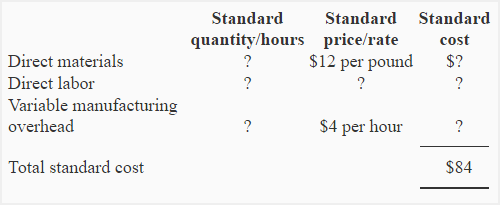During the month of May, 900 direct labor hours were actually worked and 500 units of product K were manufactured. The actual cost per unit manufactured during May was \$0.28 higher than the standard cost.

The following costs and variances information relates to the month of May: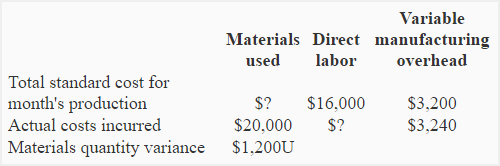Required: Compute the followings:

1. Total standard cost of materials used for actual production during May.
2. Standard quantity of materials per unit of product K.
3. Materials price variance for May.
4. Standard direct labor rate per hour.
5. Direct labor rate and efficiency variance.
6. Variable overhead spending and efficiency variance.

## Solution:

### (1) Standard cost of materials used:*\$84.00 standard cost per unit × 500 units

### (2) Standard quantity of materials per unit: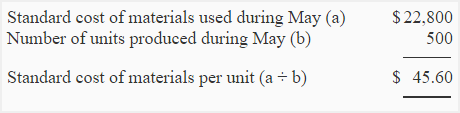Standard quantity of materials per unit = Standard cost of materials per unit/Standard cost of materials per pound

= \$45.60/\$12

= 3.8 pound per unit

### (3) Direct materials price variance: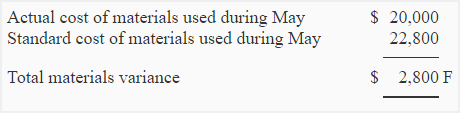Total materials variance is made up of materials price variance and materials quantity variance. Total materials variance is \$2,800 favorable and materials quantity variance is \$1,200 unfavorable. Therefore, the materials price variance must be \$4,000 as computed below: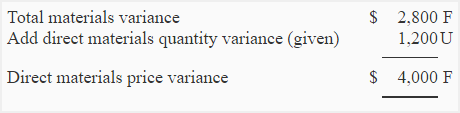### (4) Standard direct labor rate per hour:

In order to compute the standard direct labor rate per hour we need to compute the standard direct labor hours first. Since variable manufacturing overhead cost is based on direct labor hours, we can compute standard direct labor hours as follows: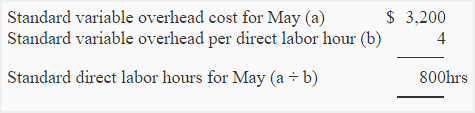Standard direct labor rate per hour = Total standard direct labor for May/Total standard direct labor hours for May

= \$16,000/800 hours

= \$20 per direct labor hour

### (5) Direct labor variances:

a. Labor rate variance:

(Actual hours worked × Actual direct labor rate) – (Actual hours worked × Standard direct labor rate)

= 18,900* – (900 hours × \$20**)

= \$18,900 – \$18,000

= \$900 Unfavorable

* Total actual cost – (Actual materials cost + Actual variable overhead cost)

= 500 units × \$84.28 – (\$20,000 + 3,240)

= 42,140 – 23,240

= \$18,900

**See requirement 4

b. Labor efficiency variance:

(Actual hours worked × Standard direct labor rate) – (Standard hours allowed × Standard direct labor rate)

= (900 hours × \$20) – (*800 hours × \$20)

= \$18,000 – \$16,000

= \$2,000 Unfavorable

*See requirement 4

### (6) Variable overhead variances:

(Actual hours worked × Actual variable overhead rate) – (Actual hours worked × Standard variable overhead rate)

= \$3,240 – (900 hours × \$4)

=\$3,240 – \$3,600

= \$360 Favorable

(Actual hours worked × Standard variable overhead rate) – (Standard hours allowed × Standard variable overhead rate)

=\$3,600 – (800 hours × \$4)

= \$3,600 – \$3,200

= \$400 Unfavorable

Show your love for us by sharing our contents.

### 3 Comments on Problem-5 (Comprehensive variance analysis, complex computations)

1.ALLLY MAKONGORO

A well illustration and clearly solutions gave me a wide coverage on prescribed topics

2.Qudratullah

Great work, thanks a lot.

3.Giovanni

Thank you, I really understand.